Sergey Horban is a QA Engineer with 3+ years of experience in automation and manual testing, bug-tracking and test management.

Become a JMeter and Continuous Testing Pro

Start Learning

## Test Your Website Performance NOW!Please enter a URL with http(s)
Jan 04 2018

# Using Operations on Variables in JMeter

In scripting, logical operations and operations of relation and comparison, are operations on variables that their result is the value "true" or "false". One of the widely used examples for applying these operations during testing is the comparison of the expected variable result with the actual variable result. This blog post will show you how to use comparison operations,  relational operations and logical operations, in your Apache JMeter™ test scripts.

Let’s get started.

# Comparison Operations

The table below shows possible comparison operations.

 Operator Description < Less If the value to the left of the operator "<" is less than the value on the right, then the result is a logical value of "TRUE" otherwise "FALSE" > More If the value to the left of the operator ">" is more than the value on the right, then the result is a logical value of "TRUE" otherwise "FALSE" <= Less or equal If the value to the left of the operator "<=" is less than or equal to the value on the right, the result is the logical value "TRUE", otherwise "FALSE" >= More or equal If the value to the left of the operator ">=" is more than or equal to the value on the right, the result is the logical value "TRUE", otherwise "FALSE

## Options for using the comparison operators >, <, <=, > =

• Comparison of a variable with a specified value
• Comparison of the value of a variable with the result of any expression
• Comparison of the results of expressions

To demonstrate the application of these operations, imagine that we have an API. In the response of this API there are the following 4 parameters with values:

• param_1 = 10;
• param_2 = 15
• param_3 = 20
• param_4 = 25

Suppose that for these parameters the following requirements exist:

• #1. The value of the parameter “param_1” should not be less than 9
• #2. The value of the parameter “param_2” should not exceed 16
• #3. The value of the parameter “param_3” must be not less than and not equal to 19
• #4. The value of the parameter “param_4” must not be greater than or equal to 26

To verify that the API returns the values according to the requirements, do the following in JMeter: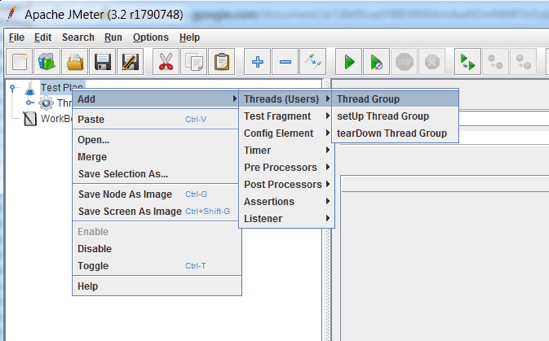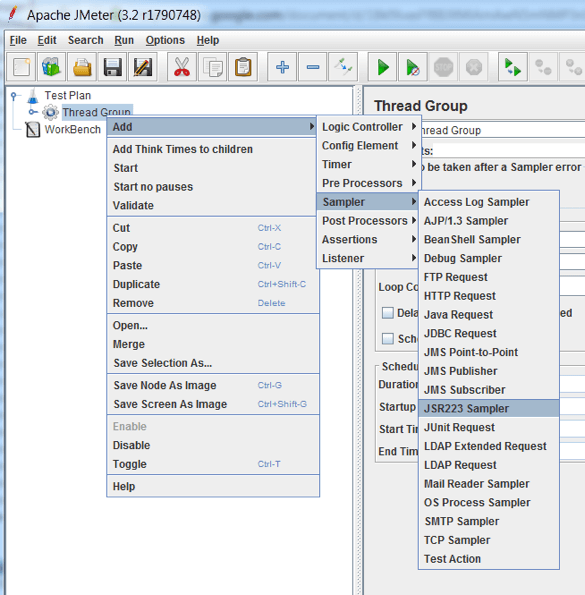3. In the JSR223 Sampler choose a language. We recommend using Groovy.

4. Open the JMeter console. The JMeter console will be used to display the code output.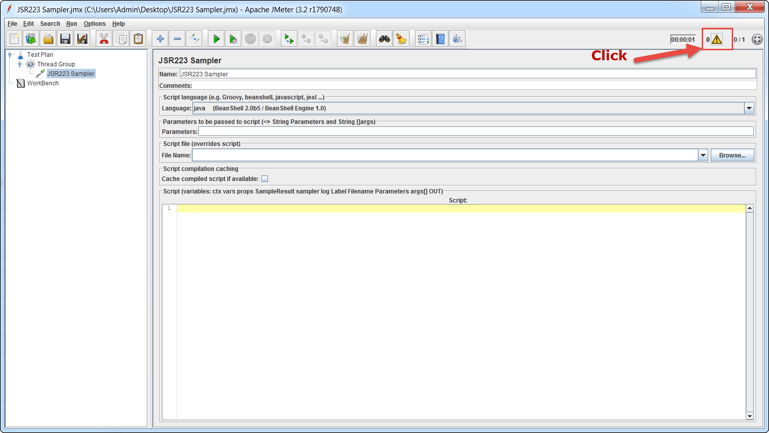5. In the JSR223 Sampler, add the following code example:

## Example code for < > <= >=

```setStrictJava (true);

int param_1 = 10;
int param_2 = 14;
int param_3 = 20;
int param_4 = 25;

if (param_1 < 9) {
log.info("The value of the param_1 does not meet the requirement #1");
} else {
log.info("The value of the param_1 meets to requirement #1");}

if (param_2 > 16) {
log.info("The value of the param_2 does not meet the requirement #2");
} else {
log.info("The value of the param_2 meets to requirement #2");
}

if (param_3 <= 19) {
log.info("The value of the param_3 does not meet the requirement #3");
} else {
log.info("The value of the param_3 meets to requirement #3");
}

if (param_4 >= 26) {
log.info("The value of the param_4 does not meet the requirement #4");
} else {
log.info("The value of the param_4 meets to requirement #4");
}
```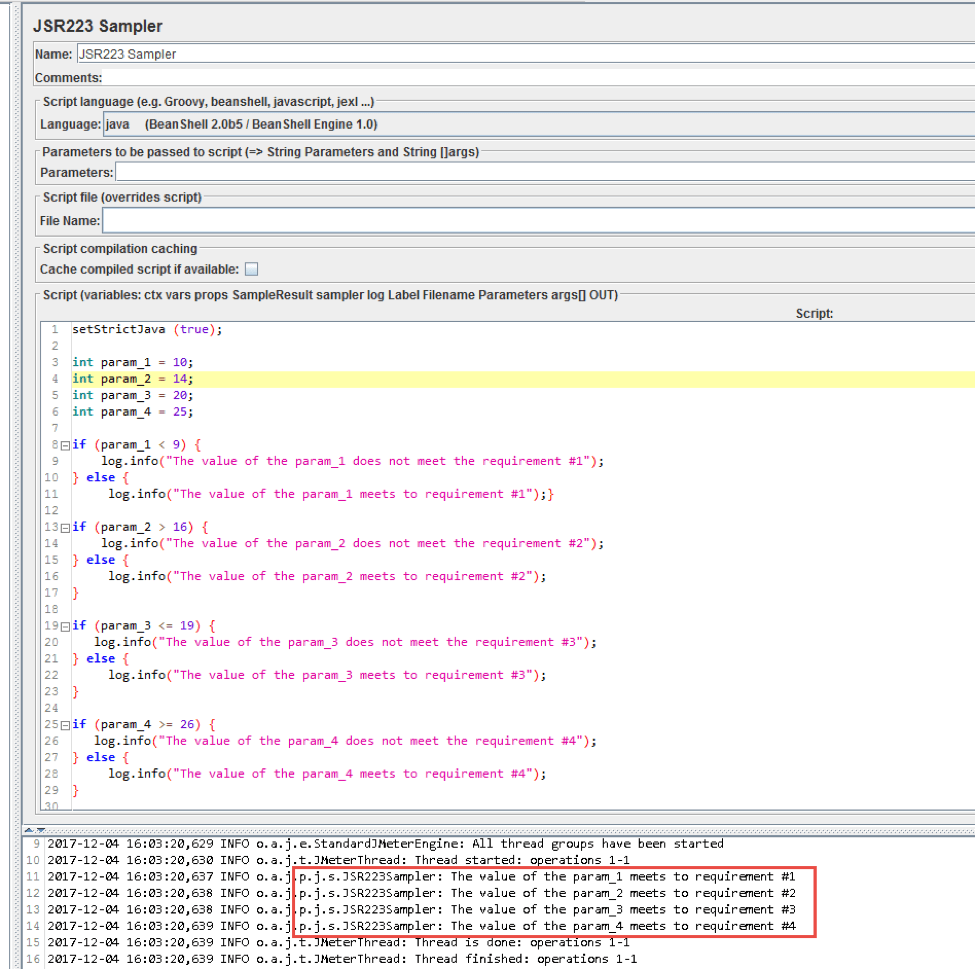In the console, you can see that the values in the API response correspond to the requirements.

Now let’s discuss the code in more detail:

param_1 - param_4 - these are parameter values that we get from the API response. In our case, these are variables with data type int.

if (condition) {  }  else {  } - This is a conditional statement that works as follows: if the “condition” is executed in parentheses, then the code is executed in curly brackets after the "if" statement. Otherwise, the code is executed in curly braces after the "else". In our case, "condition" is param_1 <9; param_2> 16; param_3 <= 19; param_4> = 26.

log.info() - A method that prints data to the JMeter console

if (param_1 < 9) {
log.info("The value of the param_1 does not meet the requirement #1");
} else {
log.info("The value of the param_1 meets to requirement #1");

If (the operator "if") the value of param_1 (in our case 10) is less than (<) the value of 9, then the requirement # 1 for the API is not executed and a message is output to the JMeter console of the following content: "The value of the param_1 does not meet the requirement # 1" , else (the operator "else") displays the message "The value of the param_1 meets to requirement # 1"

if (param_2 > 16) {
log.info("The value of the param_2 does not meet the requirement #2");
} else {
log.info("The value of the param_2 meets to requirement #2");
}

If (the operator "if") the value of param_2 (in our case 14) is more than (>) the value of 16, then the requirement # 2 for the API is not executed and a message is output to the JMeter console of the following content: "The value of the param_2 does not meet the requirement # 2" , else (the operator "else") displays the message "The value of the param_2 meets to requirement # 2"

if (param_3 <= 19) {
log.info("The value of the param_3 does not meet the requirement #3");
} else {
log.info("The value of the param_3 meets to requirement #3");
}

If (the operator "if") the value of param_3 (in our case 20) is less than the value 19 or equal to the value 19 (<=), then the requirement # 3 for the API is not executed and a message is output to the JMeter console of the following content: "The value of the param_3 does not meet the requirement # 3" , else (the operator "else") displays the message "The value of the param_3 meets to requirement # 3"

if (param_4 >= 26) {
log.info("The value of the param_4 does not meet the requirement #4");
} else {
log.info("The value of the param_4 meets to requirement #4");
}

If (the operator "if") the value of param_4 (in our case 25) is more than the value 26 or equal to the value 26 (>=), then the requirement # 4 for the API is not executed and a message is output to the JMeter console of the following content: "The value of the param_4 does not meet the requirement # 4" , else (the operator "else") displays the message "The value of the param_4 meets to requirement # 4".

# Relational Operations

Relational operations check for equality and inequality. The table below shows possible relational operations.

 Operator Description == Checks for equality. If the value to the left of the "==" operator is equal to the value on the right, the result is the logical value "TRUE", otherwise "FALSE" != Checks for inequality If the value to the left of the "!=" operator is not equal to the value on the right, the result is the logical value "TRUE", otherwise "FALSE"

## Options for using the relational operators  "==" and "! ="

• Check for equality or inequality of a variable with the specified value
• Check for equality or inequality of the value of a variable with the result of any expression
• Check for equality or inequality of expression results

## Example Code for ==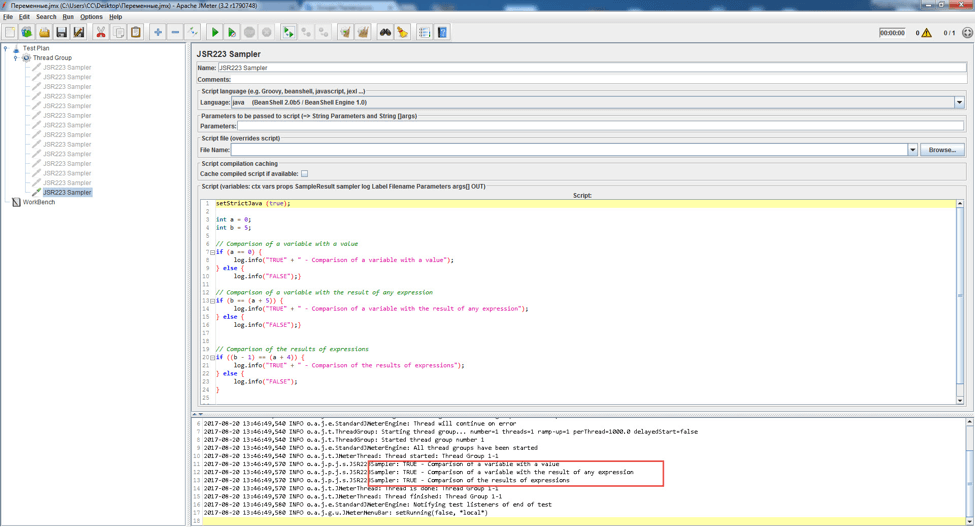```setStrictJava (true);

int a = 0;
int b = 5;

// Comparison of a variable with a value
if (a == 0) {
log.info("TRUE" + " - Comparison of a variable with a value");
} else {
log.info("FALSE");}

// Comparison of a variable with the result of any expression
if (b == (a + 5)) {
log.info("TRUE" + " - Comparison of a variable with the result of any expression");
} else {
log.info("FALSE");}

// Comparison of the results of expressions
if ((b - 1) == (a + 4)) {
log.info("TRUE" + " - Comparison of the results of expressions");
} else {
log.info("FALSE");
}
```

The code example above shows how to use the "==" operation.

In simple words, the following code can be described as follows:

if (a == 0) {
log.info("TRUE" + " - Comparison of a variable with a value");
} else {
log.info("FALSE");}

If the variable "a" equals "0", then output the following message to the JMeter console: "TRUE  - Comparison of a variable with a value", otherwise output the message "FALSE". Since in our case the variable "a" = 0, the message "TRUE  - Comparison of a variable with a value" is output to the console.

if (b == (a + 5)) {
log.info("TRUE" + " - Comparison of a variable with the result of any expression");
} else {
log.info("FALSE");}

If the variable "b" is equal to the sum of the variable "a" and 5, then output the following message to the console: "TRUE - Comparison of a variable with the result of any expression", otherwise output the message "FALSE".

In the expression b == (a + 5), the addition operation "+" is performed first, and then the comparison operation "==". In our case, the variable "b" = 5, the sum of a + 5 = 5, since the variable "a" = 0 and from this the message "TRUE - Comparison of a variable with the result of any expression" is output to the console.

if ((b - 1) == (a + 4)) {
log.info("TRUE" + " - Comparison of the results of expressions");
} else {
log.info("FALSE");
}

If the difference between the variable "b" and 1 will equal the sum of the variable "a" and 4, then output the following message to the console "TRUE - Comparison of the results of expressions", otherwise output the message "FALSE".

In the expression (b - 1) == (a + 4), the "-" subtraction operation is performed first, then the addition operation "+", and then the comparison operation "==". In our case, the variable "b" = 5 (b - 1 = 4), the variable "a" = 0 (а + 4 = 4), since 4 = 4 the message  "TRUE - Comparison of the results of expressions" outputs.

## Example code for !=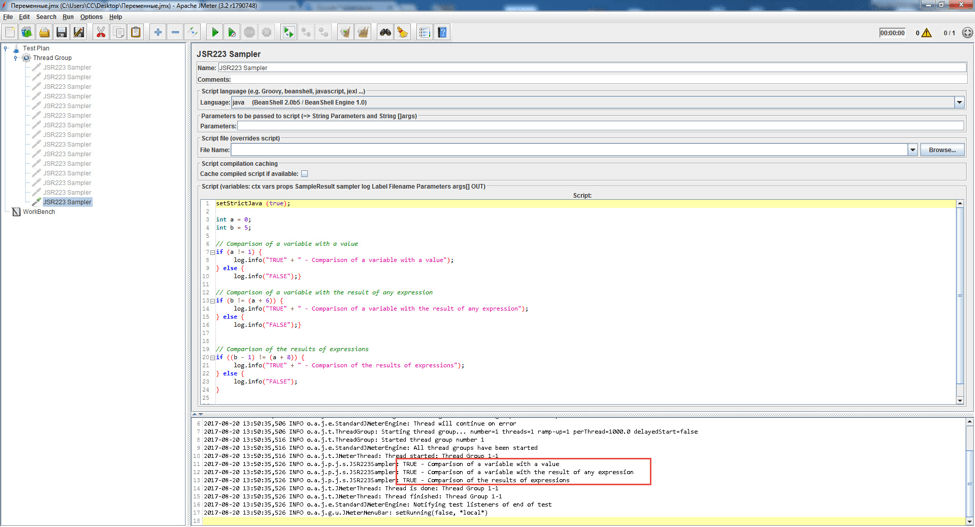```setStrictJava (true);

int a = 0;
int b = 5;

// Comparison of a variable with a value
if (a != 1) {
log.info("TRUE" + " - Comparison of a variable with a value");
} else {
log.info("FALSE");}

// Comparison of a variable with the result of any expression
if (b != (a + 6)) {
log.info("TRUE" + " - Comparison of a variable with the result of any expression");
} else {
log.info("FALSE");}

// Comparison of the results of expressions
if ((b - 1) != (a + 8)) {
log.info("TRUE" + " - Comparison of the results of expressions");
} else {
log.info("FALSE");
}
```

The code example above shows how to use the "!=" operation. Let's analyze in more detail the most voluminous part of the code, since its understanding will make it possible to understand how the operation "! =" works.

In simple words, the following code can be described as follows:

if ((b - 1) != (a + 8)) {
log.info("TRUE" + " - Comparison of the results of expressions");
} else {
log.info("FALSE");
}

If the difference between the variable "b" and 1 will not equal the sum of the variable "a" and 8, then output the following message to the console "TRUE - Comparison of the results of expressions", otherwise output the message "FALSE".

In the expression (b - 1) != (a + 8), the "-" subtraction operation is performed first, then the addition operation "+", and then the inequality operation "!=". In our case, the variable "b" = 5 (b - 1 = 4), the variable "a" = 0 (а + 8 = 8), since 4 does not equal 8, the message  "TRUE - Comparison of the results of expressions" is sent to the console.

# Logical Operations

 Operator Description && Logical operation "AND" If the result of evaluating expressions that are to the left and right of && is "TRUE", the && operation returns "TRUE" If the result of the calculation of at least one expression to the left or right of the operator && returns the result "FALSE", the operation && returns the value "FALSE" An expression for the operation "&&" can be a variable with a data type boolean || The logical operation "OR" If the result of evaluating at least one expression on the left or right of the operation || Returns the result "TRUE", then the operation || Returns "TRUE" If the result of evaluating the expression to the left of the operation || Is equal to "TRUE", then the expression to the right of the operation || Will not be calculated An expression for the operation "||" can be a variable with a data type boolean ! Logical operation "denial" or "NOT" If the actual result of evaluating the expression (for example a + 1 == 2) is a logical value of "TRUE", then the operation! Returns the logical value "TRUE" only if the actual value of the expression a + 1 is not 2

## Options for using the operators "&&", "||", "!"

• To execute the logic of the code on the given branches, depending on the truth or falsity of the calculation result of the operation "&&", "||", "!".

## Example code for &&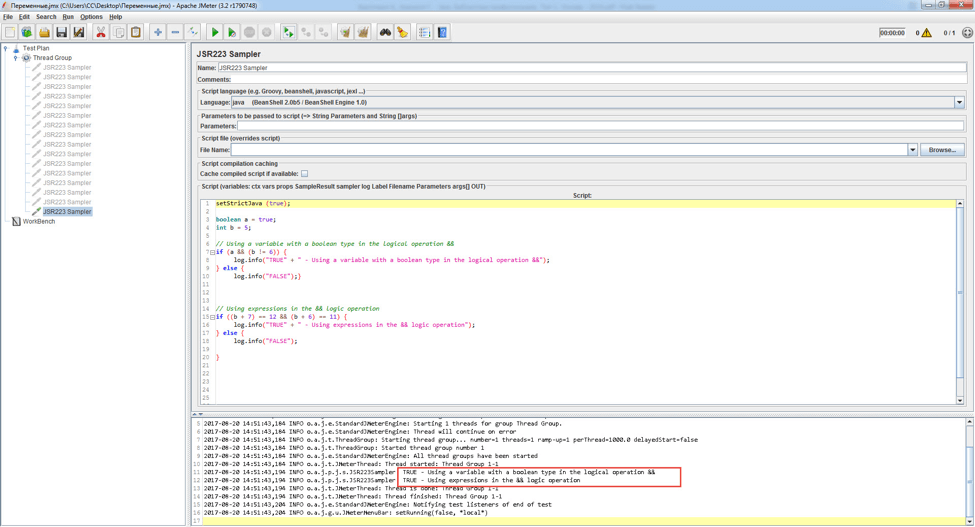```setStrictJava (true);

boolean a = true;
int b = 5;

// Using a variable with a boolean type in the logical operation &&
if (a && (b != 6)) {
log.info("TRUE" + " - Using a variable with a boolean type in the logical operation &&");
} else {
log.info("FALSE");}

// Using expressions in the && logic operation
if ((b + 7) == 12 && (b + 6) == 11) {
log.info("TRUE" + " - Using expressions in the && logic operation");
} else {
log.info("FALSE");

}
```

The code example above shows how to use the "&&" operation. Let's analyze in more detail the most voluminous part of the code, its understanding will make it possible to understand how the operation "&&".

In simple words, the following code can be described as follows:

if ((b + 7) == 12 && (b + 6) == 11) {
log.info("TRUE" + " - Using expressions in the && logic operation");
} else {
log.info("FALSE");

}

If the sum of the variable "b" and 7 will equal 12 and the sum of the variable "b" and 6 will equal 11, then output to the console the following message "TRUE  - Using expressions in the && logic operation", otherwise output the message " FALSE".

In the expression (b + 7) == 12 && (b + 6) == 11, the addition operation b + 7 is performed first, then the comparison operation "==", if b + 7 will equal 12, then proceed with the operation (b + 6) == 11. If b + 7 will not equal to 12, then the operation (b + 6) == 11 will not be executed and the message "FALSE" will be displayed in the console.

In our case, the variable "b" = 5 (5 + 7 = 12), (5 + 6 = 11), since the conditions on the left and right of the "&&" operator are fulfilled, the "TRUE  - Using expressions in the && logic operation" message is output to the console.

## Example code for ||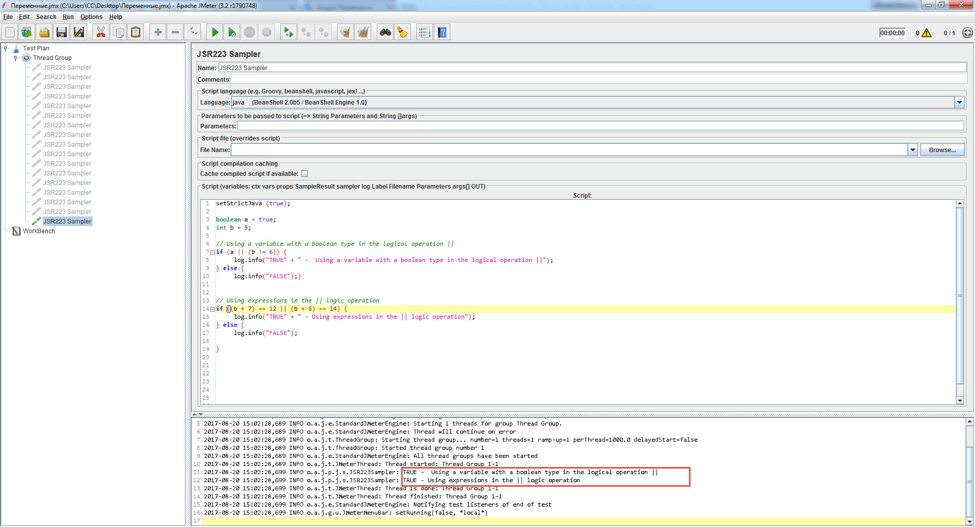```setStrictJava (true);

boolean a = true;
int b = 5;

// Using a variable with a boolean type in the logical operation ||
if (a || (b != 6)) {
log.info("TRUE" + " -  Using a variable with a boolean type in the logical operation ||");
} else {
log.info("FALSE");}

// Using expressions in the || logic operation
if ((b + 7) == 12 || (b + 6) == 14) {
log.info("TRUE" + " - Using expressions in the || logic operation");
} else {
log.info("FALSE");

}
```

The code example above shows how you can use the operation "||". This operation is used when only one of the several conditions must be fulfilled. It is not often used in testing, but sometimes there is a need for this operation.

In simple words, this code can be described as follows:

if (a || (b != 6)) {
log.info("TRUE" + " -  Using a variable with a boolean type in the logical operation ||");
} else {
log.info("FALSE");}

If the variable “a” has a logical value of "true" or the variable “b” is not 6, then output to the console a message. In our case, the variable a = true, then the message "TRUE -  Using a variable with a boolean type in the logical operation || " is output to the console.  If you change the value of the variable to a = false, then the message is also output to the console, since the condition b ! = 6 is fulfilled

## Example code for !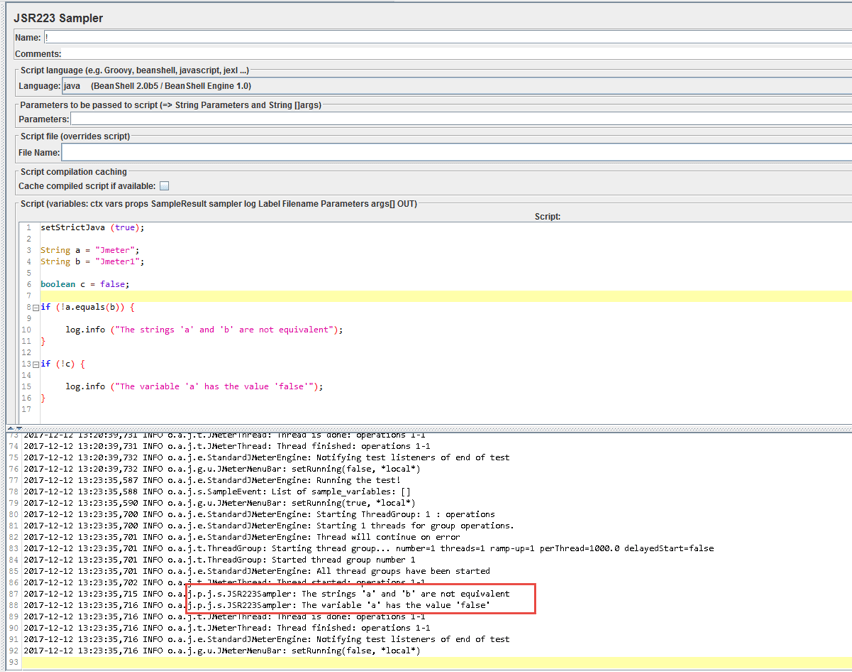```setStrictJava (true);

String a = "Jmeter";
String b = "Jmeter1";

boolean c = false;

if (!a.equals(b)) {

log.info ("The strings 'a' and 'b' are not equivalent");
}

if (!c) {

log.info ("The variable 'a' has the value 'false'");}
```

The code example above shows how to use the "!" operation.

In simple words, the following code can be described as follows:

if (!a.equals(b)) {

log.info ("The strings 'a' and 'b' are not equivalent");
}

If strings  ‘a’ and ‘b’ are not equivalent, then output the following message to JMeter console: "The strings 'a' and 'b' are not equivalent". In our case, ‘a’ and ‘b’ are not equivalent, so the message is output to the console

if (!c) {

log.info ("The variable 'a' has the value 'false'");
}

If the value of the variable ‘c’ is not "true", then output the following message to the JMeter console: "The variable 'a' has the value 'false". In our case, c = false, then the message is output to the console.Please enter a URL with http(s)